# Arduino programs Arduino toolchain Crosscompilation Arduino sketches Classes

• Slides: 36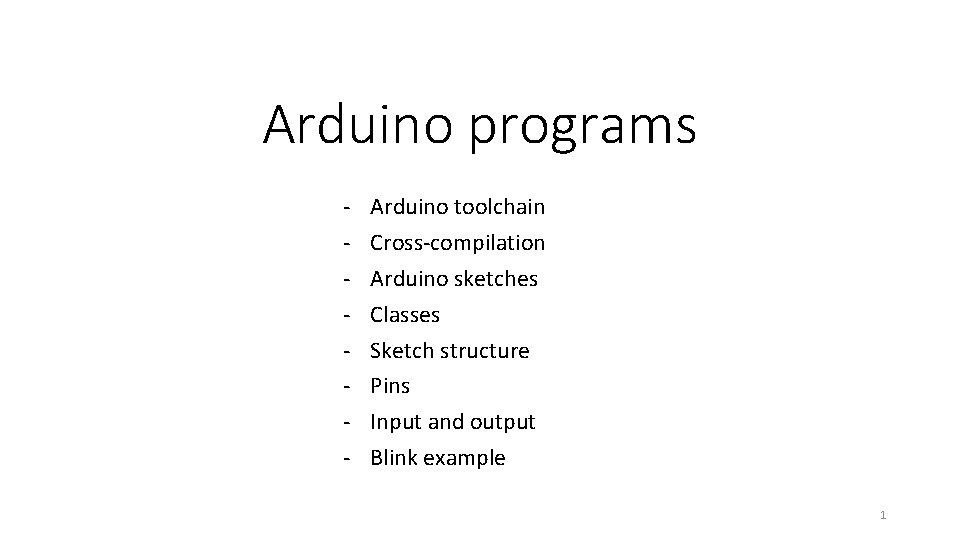Arduino programs - Arduino toolchain Cross-compilation Arduino sketches Classes Sketch structure Pins Input and output Blink example 1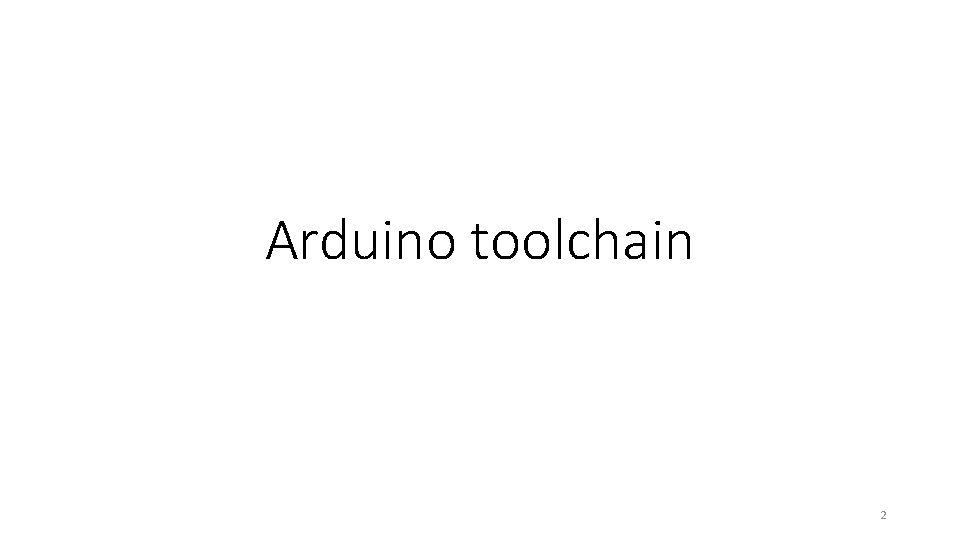Arduino toolchain 2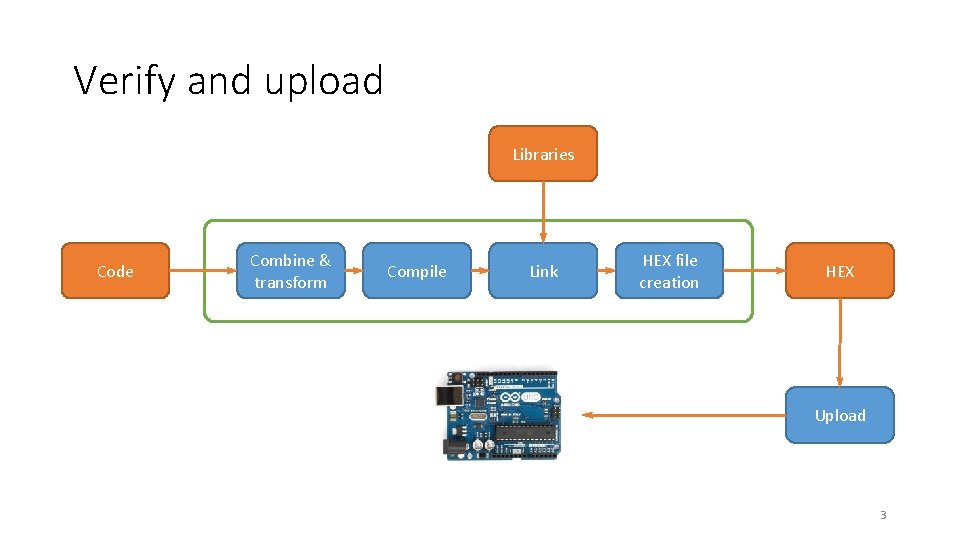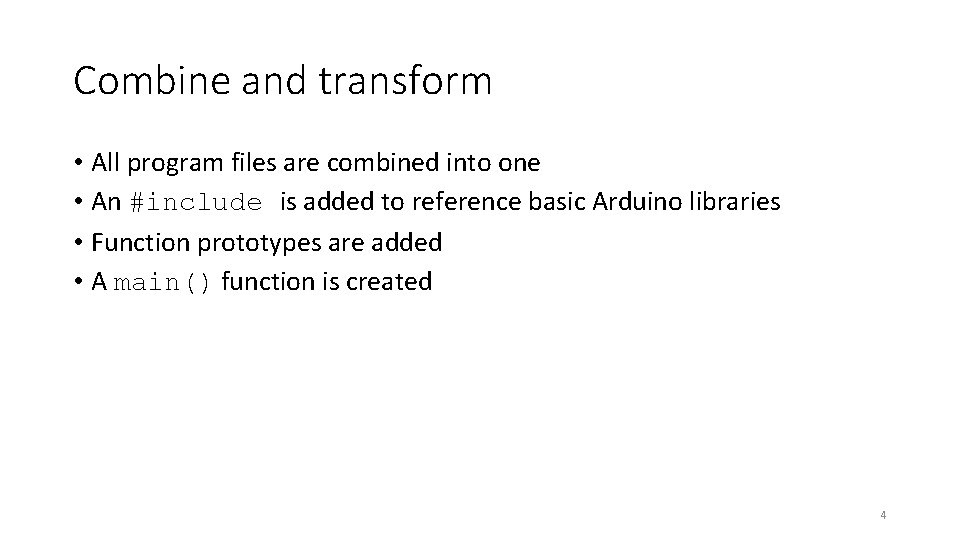Combine and transform • All program files are combined into one • An #include is added to reference basic Arduino libraries • Function prototypes are added • A main() function is created 4Cross-compilation 5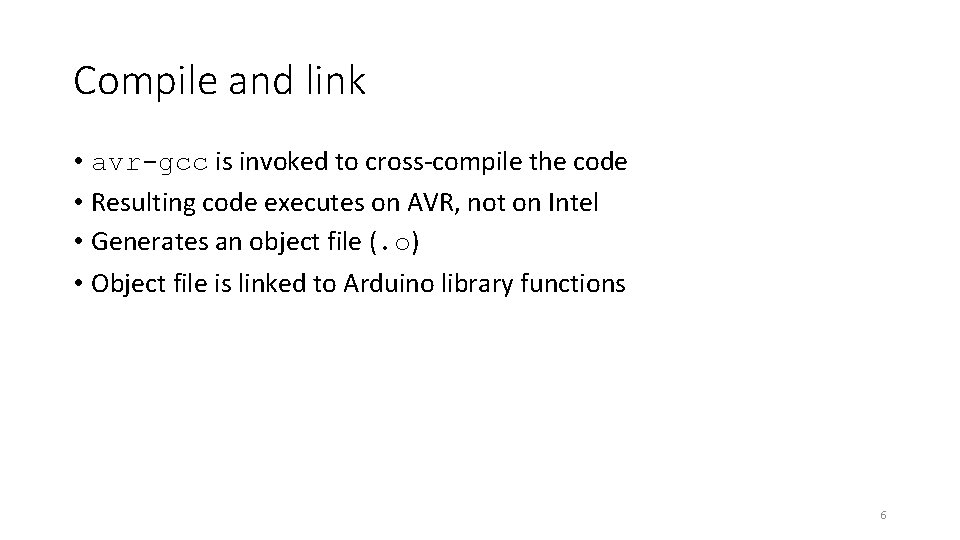Compile and link • avr-gcc is invoked to cross-compile the code • Resulting code executes on AVR, not on Intel • Generates an object file (. o) • Object file is linked to Arduino library functions 6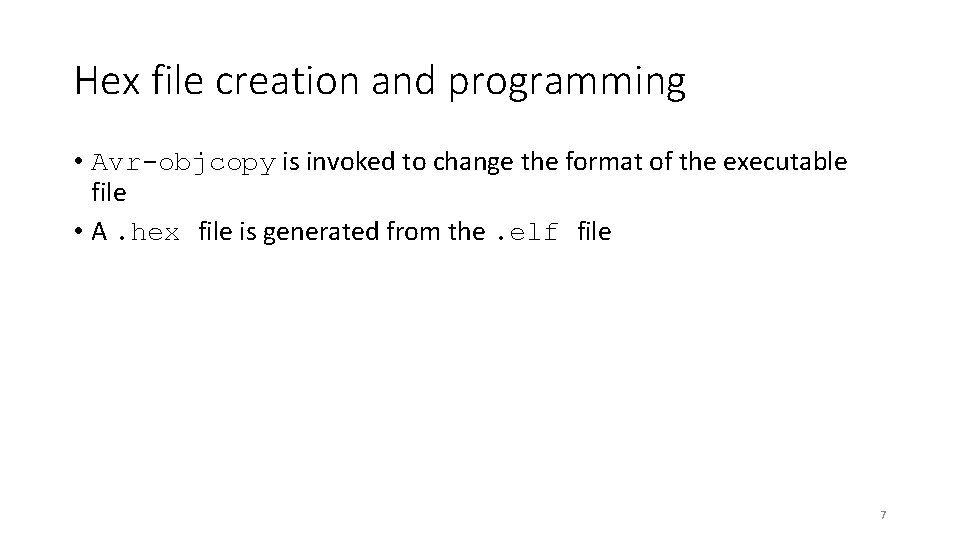Hex file creation and programming • Avr-objcopy is invoked to change the format of the executable file • A. hex file is generated from the. elf file 7Arduino sketches 8Arduino programs • A program is called a sketch • C++ program using Arduino library functions • C++ is a superset of C • All C programs are legal C++ • C++ also includes classes 9Object-oriented programming • Organize your code through encapsulation • Group together data and functions that are related • User-defined type is specific to an application • int type has data (the number) and functions (+, -, *) 10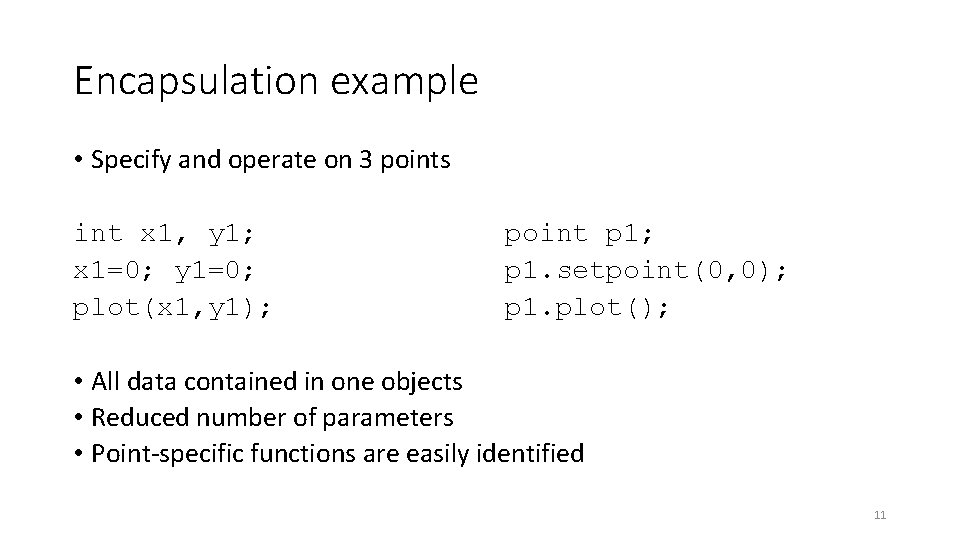Encapsulation example • Specify and operate on 3 points int x 1, y 1; x 1=0; y 1=0; plot(x 1, y 1); point p 1; p 1. setpoint(0, 0); p 1. plot(); • All data contained in one objects • Reduced number of parameters • Point-specific functions are easily identified 11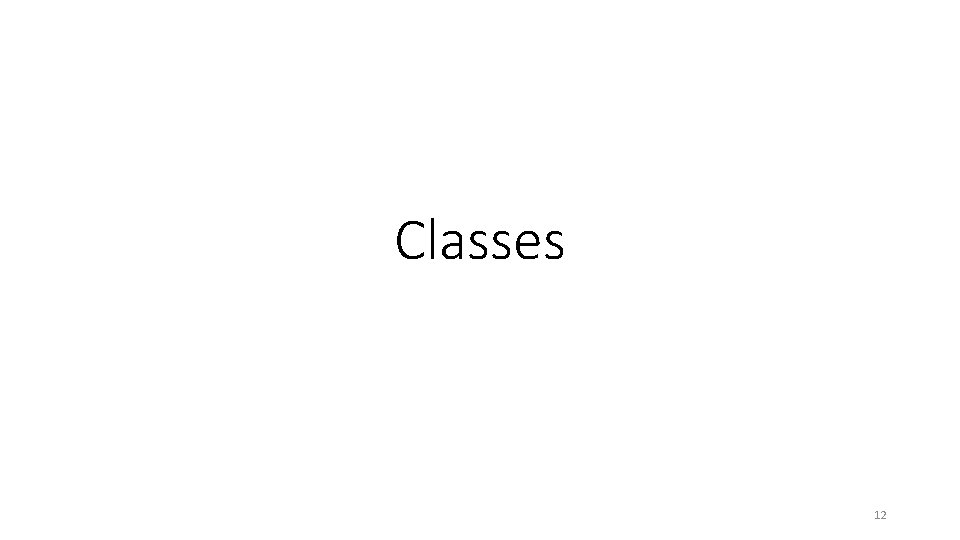Classes 12Classes and members class X { public: int m; int mf(int v){int old=m; m=v; return old; } }; X var; var. m=7; int z = var. mf(9); 13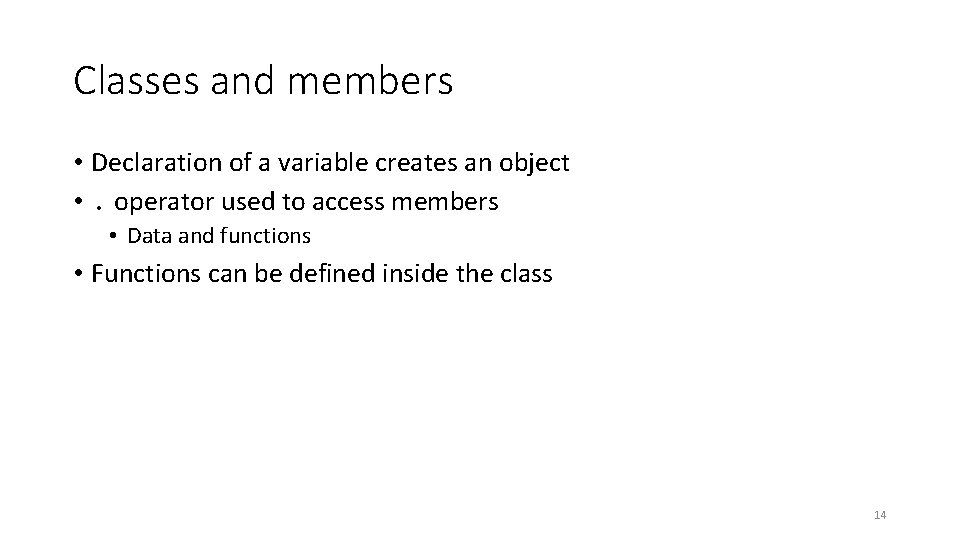Classes and members • Declaration of a variable creates an object • . operator used to access members • Data and functions • Functions can be defined inside the class 14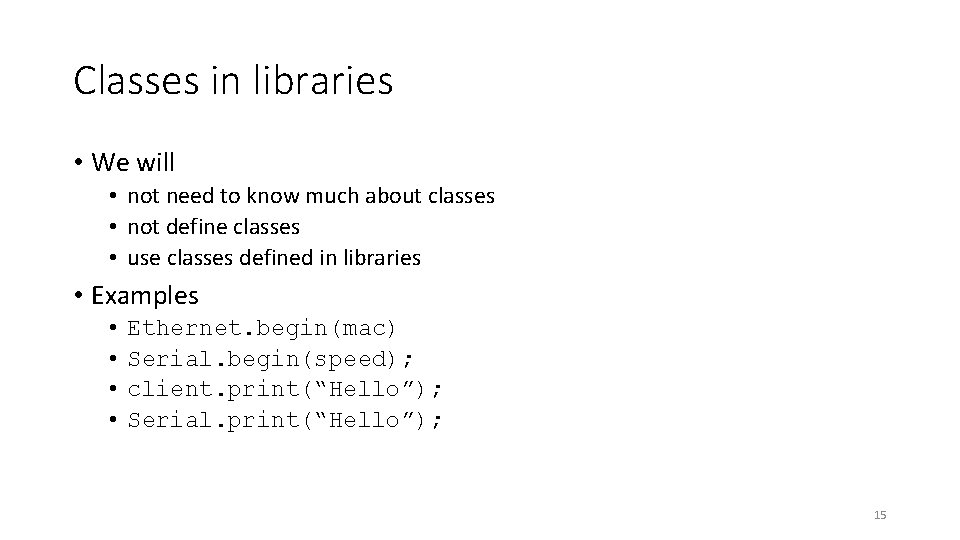Classes in libraries • We will • not need to know much about classes • not define classes • use classes defined in libraries • Examples • • Ethernet. begin(mac) Serial. begin(speed); client. print(“Hello”); Serial. print(“Hello”); 15Sketch structure 16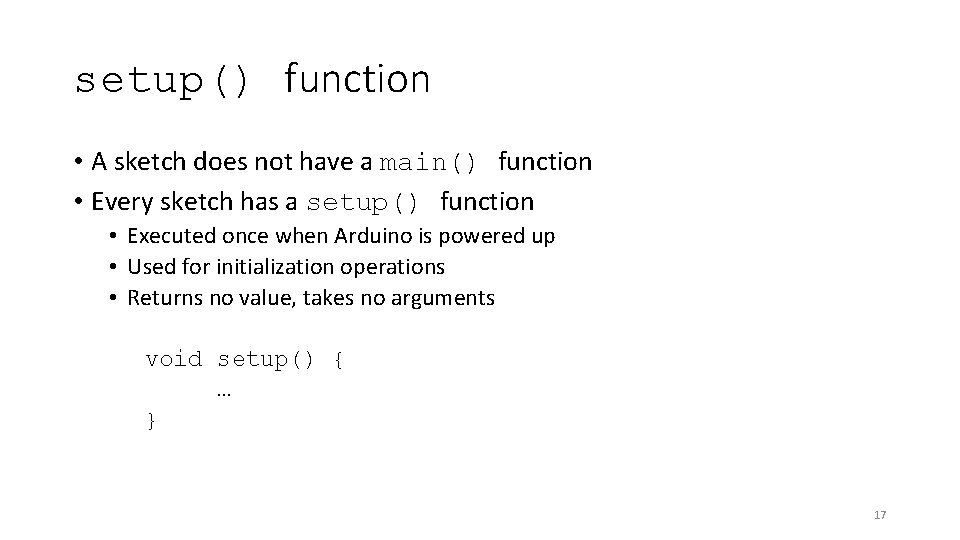setup() function • A sketch does not have a main() function • Every sketch has a setup() function • Executed once when Arduino is powered up • Used for initialization operations • Returns no value, takes no arguments void setup() { … } 17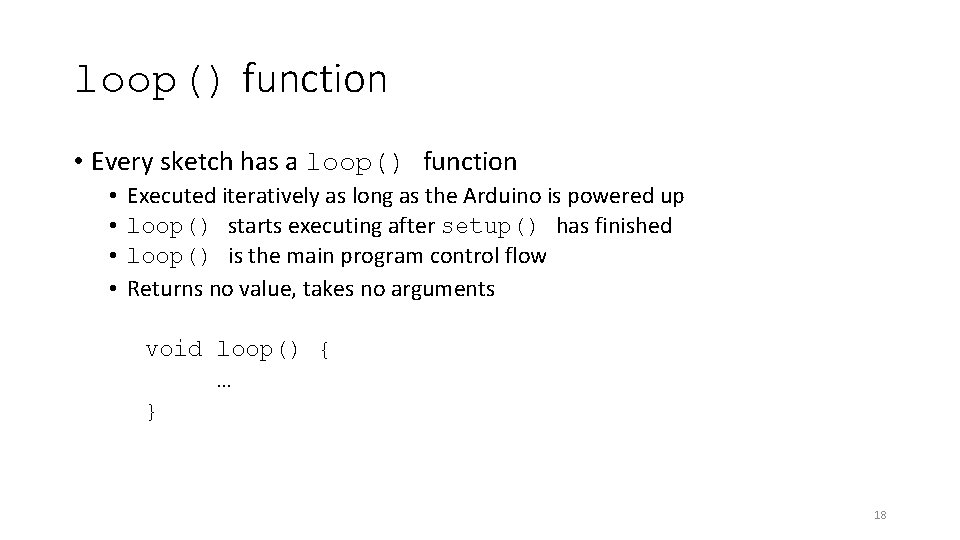loop() function • Every sketch has a loop() function • • Executed iteratively as long as the Arduino is powered up loop() starts executing after setup() has finished loop() is the main program control flow Returns no value, takes no arguments void loop() { … } 18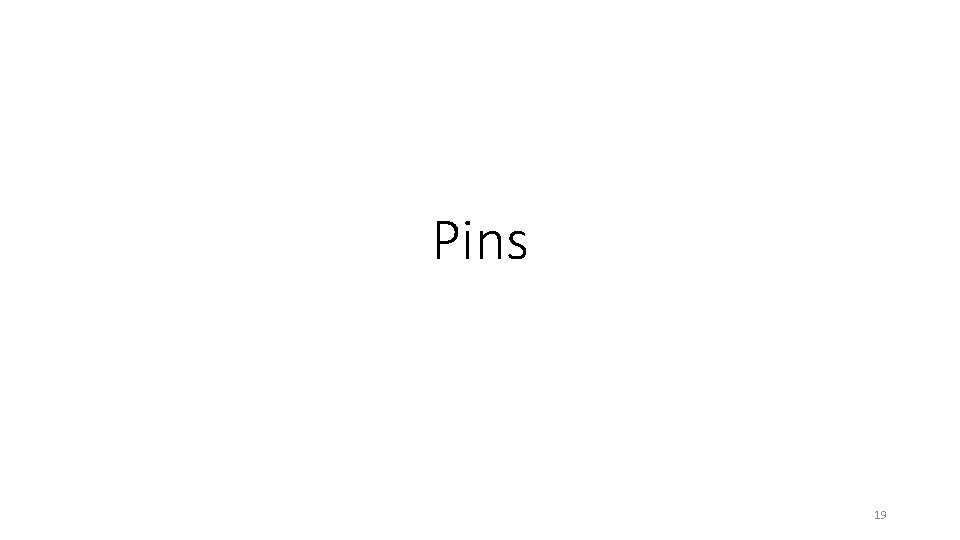Pins 19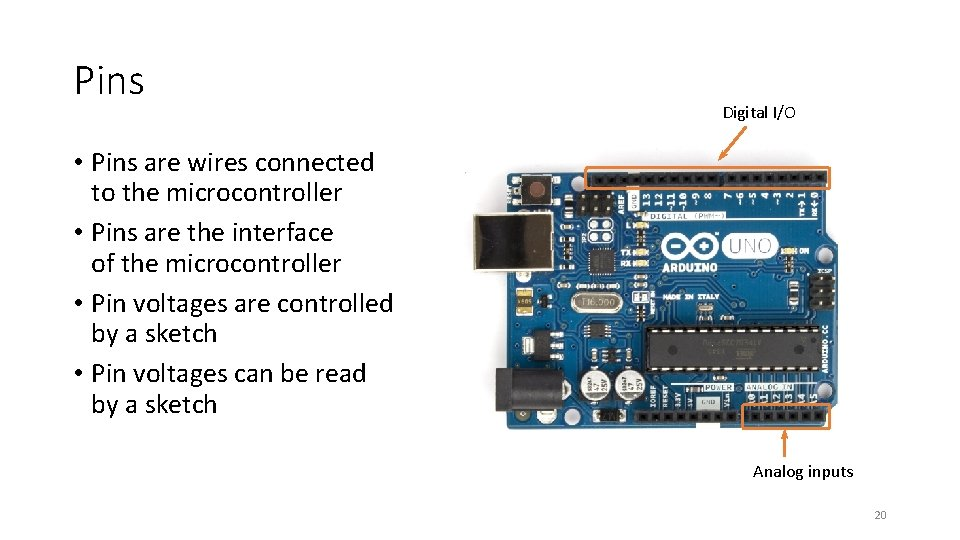Pins Digital I/O • Pins are wires connected to the microcontroller • Pins are the interface of the microcontroller • Pin voltages are controlled by a sketch • Pin voltages can be read by a sketch Analog inputs 20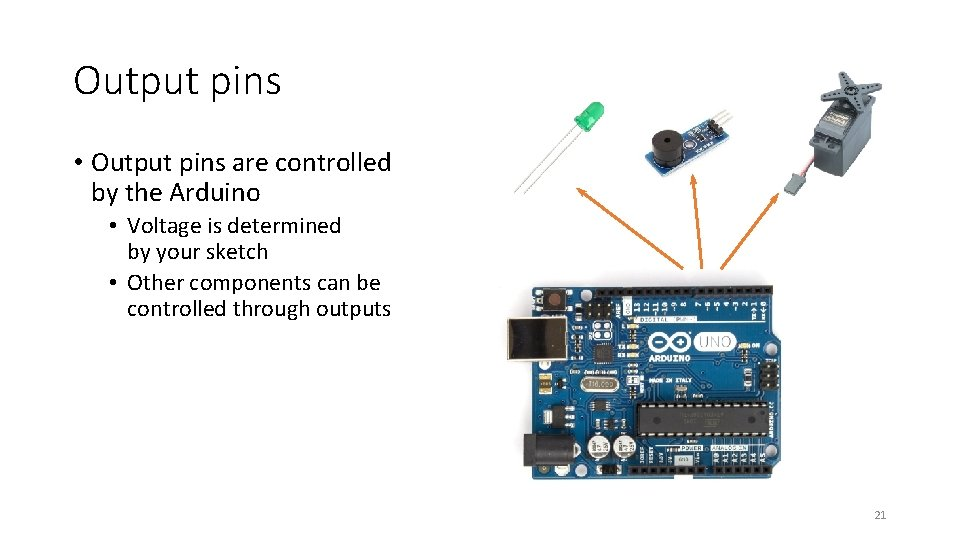Output pins • Output pins are controlled by the Arduino • Voltage is determined by your sketch • Other components can be controlled through outputs 21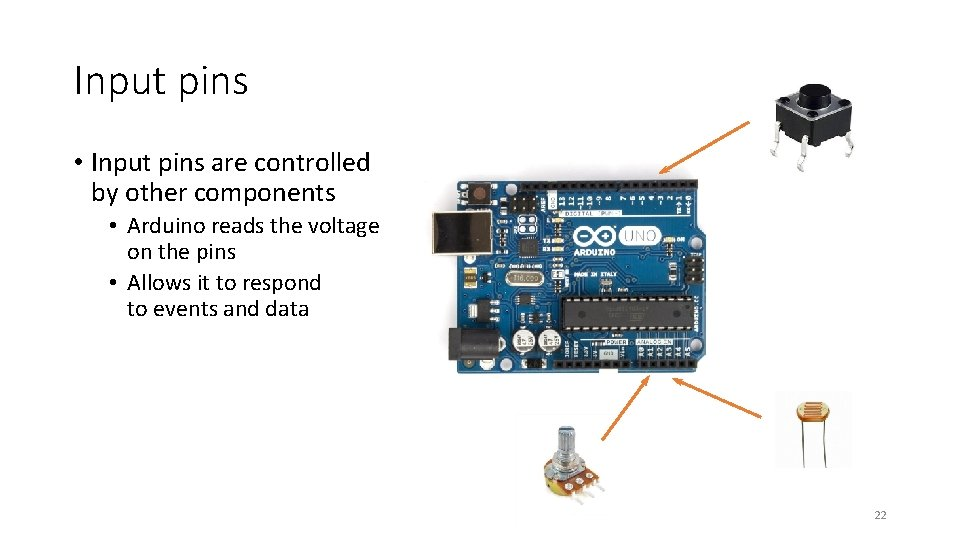Input pins • Input pins are controlled by other components • Arduino reads the voltage on the pins • Allows it to respond to events and data 22Digital vs. analog • Some pins are digital-only • Read digital input, write digital output • 0 volts or 5 volts • Some pins can be analog inputs • Can read analog voltages on the pin • Useful for analog sensors • Analog-only pins are clearly labeled • No pins can generate an analog output 23Input and output 24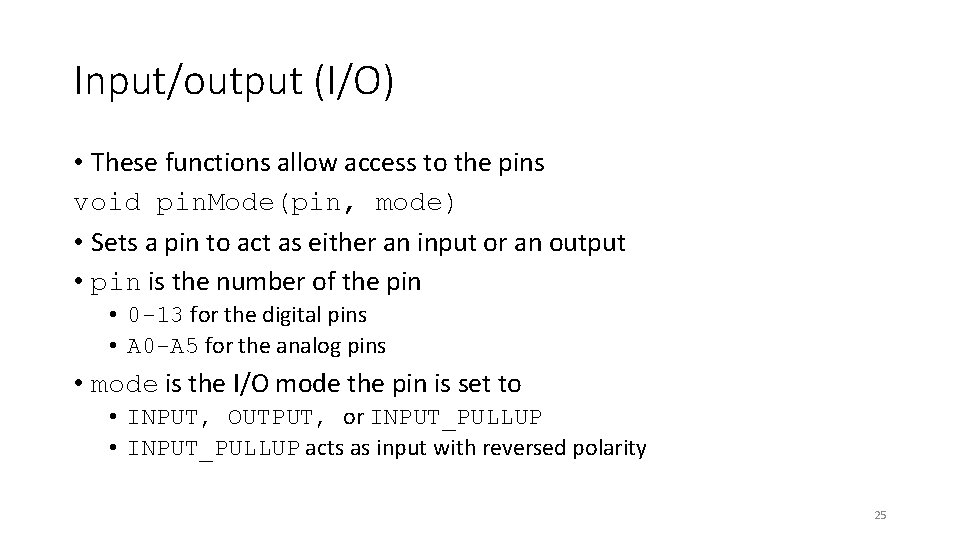Input/output (I/O) • These functions allow access to the pins void pin. Mode(pin, mode) • Sets a pin to act as either an input or an output • pin is the number of the pin • 0 -13 for the digital pins • A 0 -A 5 for the analog pins • mode is the I/O mode the pin is set to • INPUT, OUTPUT, or INPUT_PULLUP • INPUT_PULLUP acts as input with reversed polarity 25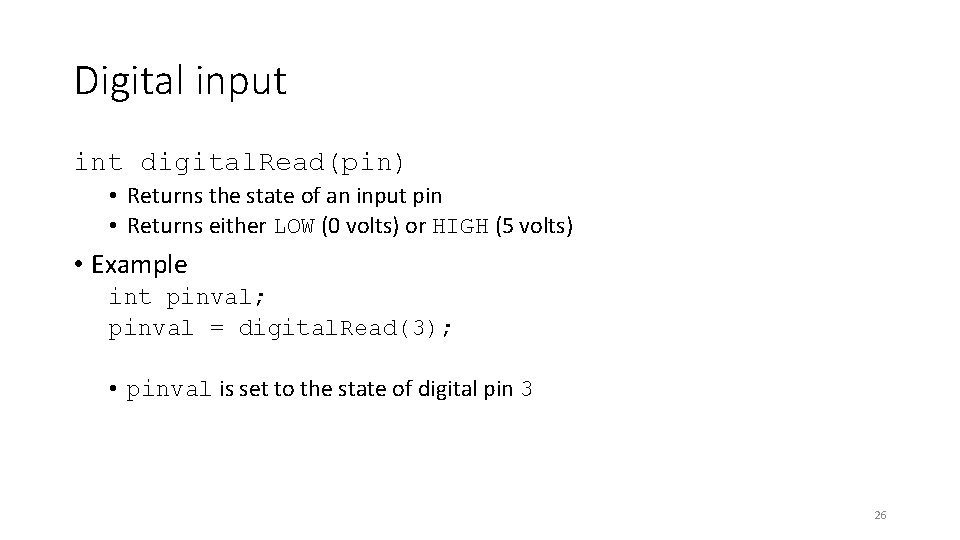Digital input int digital. Read(pin) • Returns the state of an input pin • Returns either LOW (0 volts) or HIGH (5 volts) • Example int pinval; pinval = digital. Read(3); • pinval is set to the state of digital pin 3 26Digital output void digital. Write(pin, value) • Assigns the state of an output pin • Assigns either LOW (0 volts) or HIGH (5 volts) • Example digital. Write(3, HIGH); • Digital pin 3 is set HIGH (5 volts) 27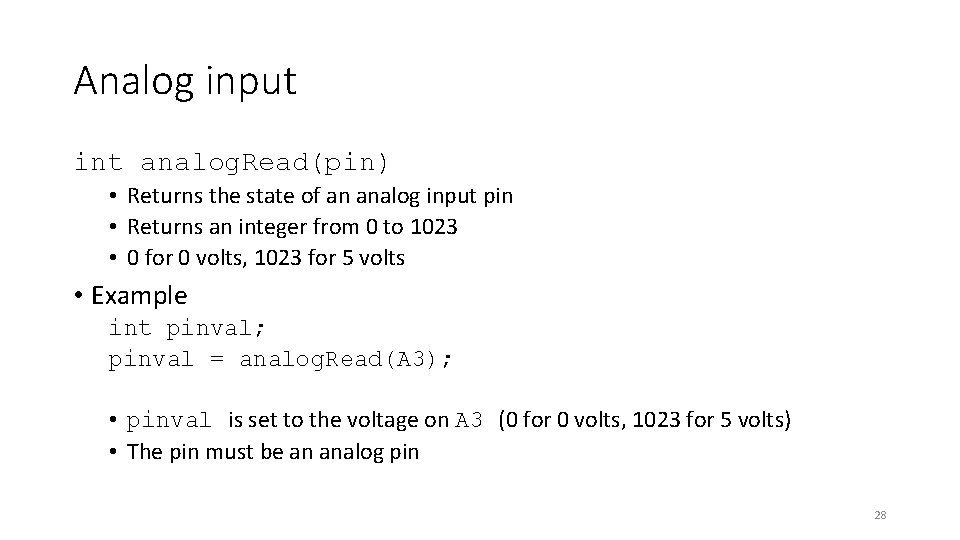Analog input int analog. Read(pin) • Returns the state of an analog input pin • Returns an integer from 0 to 1023 • 0 for 0 volts, 1023 for 5 volts • Example int pinval; pinval = analog. Read(A 3); • pinval is set to the voltage on A 3 (0 for 0 volts, 1023 for 5 volts) • The pin must be an analog pin 28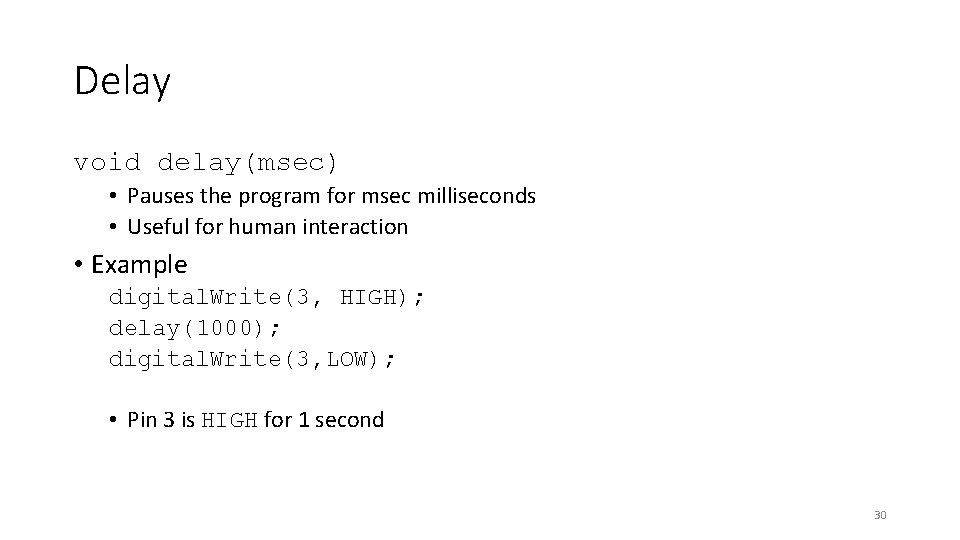Delay void delay(msec) • Pauses the program for msec milliseconds • Useful for human interaction • Example digital. Write(3, HIGH); delay(1000); digital. Write(3, LOW); • Pin 3 is HIGH for 1 second 30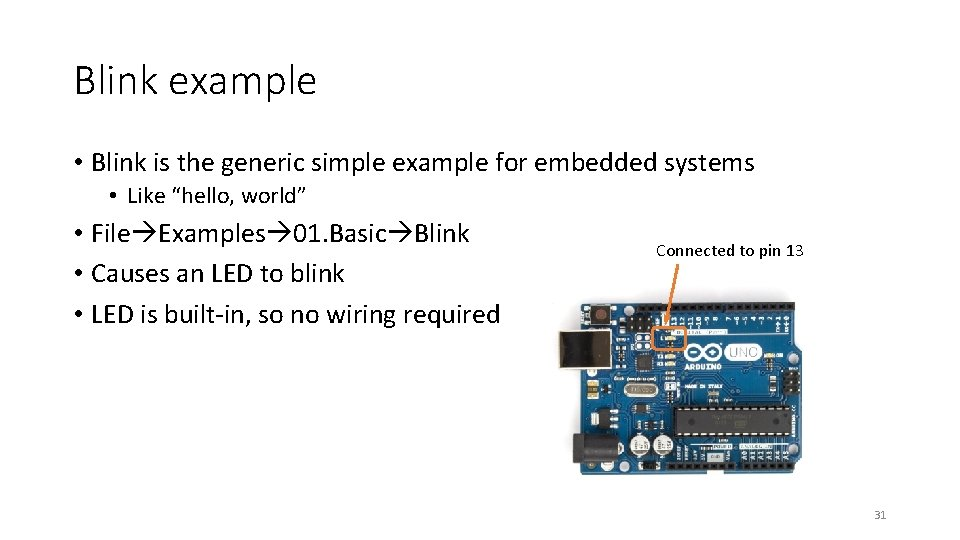Blink example • Blink is the generic simple example for embedded systems • Like “hello, world” • File Examples 01. Basic Blink • Causes an LED to blink • LED is built-in, so no wiring required Connected to pin 13 31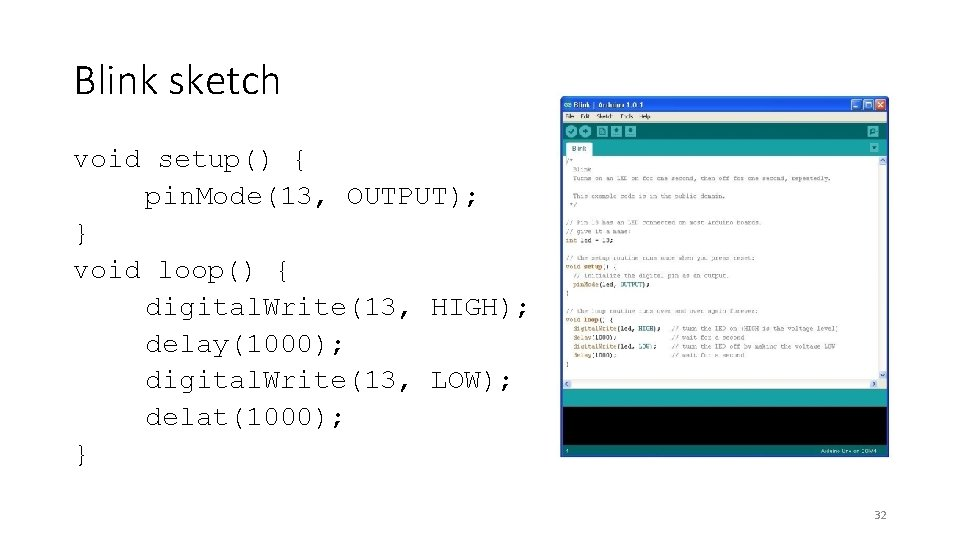Blink sketch void setup() { pin. Mode(13, OUTPUT); } void loop() { digital. Write(13, HIGH); delay(1000); digital. Write(13, LOW); delat(1000); } 32Lab 33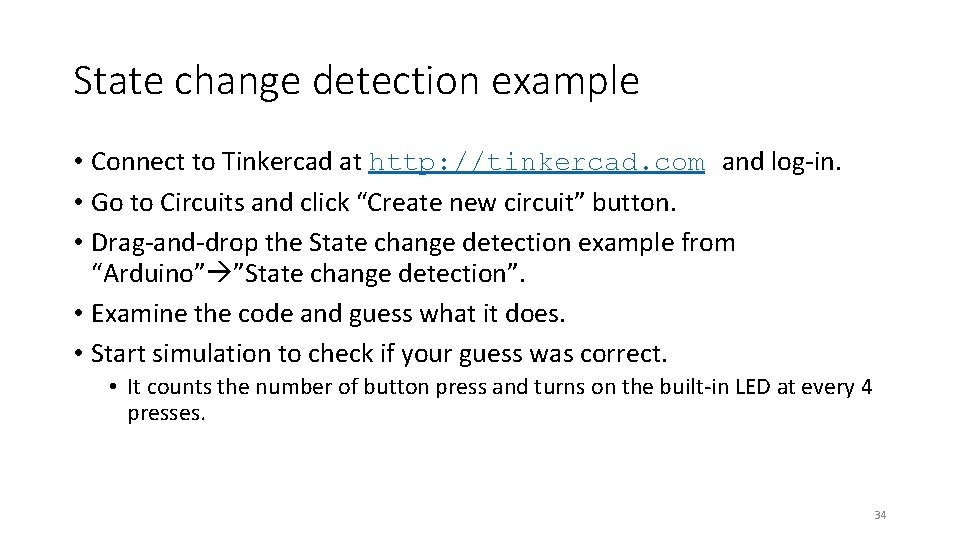State change detection example • Connect to Tinkercad at http: //tinkercad. com and log-in. • Go to Circuits and click “Create new circuit” button. • Drag-and-drop the State change detection example from “Arduino” ”State change detection”. • Examine the code and guess what it does. • Start simulation to check if your guess was correct. • It counts the number of button press and turns on the built-in LED at every 4 presses. 34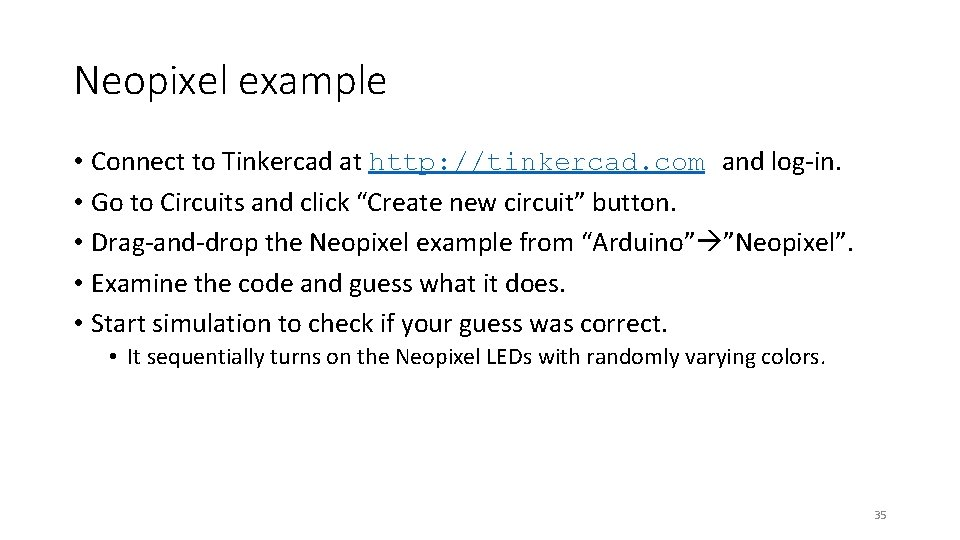Neopixel example • Connect to Tinkercad at http: //tinkercad. com and log-in. • Go to Circuits and click “Create new circuit” button. • Drag-and-drop the Neopixel example from “Arduino” ”Neopixel”. • Examine the code and guess what it does. • Start simulation to check if your guess was correct. • It sequentially turns on the Neopixel LEDs with randomly varying colors. 35Neopixel with a button • Combine “State change detection” and “Neopixel” to have your simulator do the following. • When your Arduino is turned on, the Neopixel is sequentially turned on with random colors. • Neopixel color changes when the number of button press increases (that is when the button status changes from “Released” to “Pushed”). 36